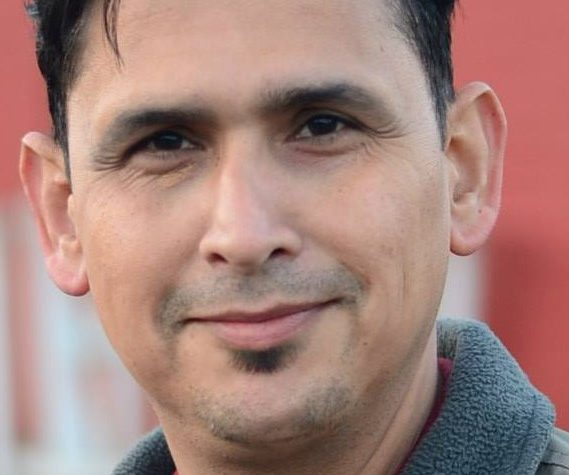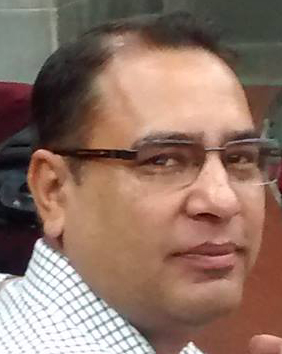April 10, 2021

Forum for KU Academics

# Perspectives of Mathematics

Kanhaiya Jha
Distinguished scientist and former Indian president Dr. A.P.J. Abdul Kalam summarizes the significance of science to the mankind thus: “Science, more science and still more science is needed to lay the foundations for the development and growth of an individual, a society, a nation and world.” Science has made invaluable contributions in the progress of different civilizations of the world. Math-e-matics has been defined as the science of numbers and the structure and measurement of shapes, including algebra, geometry and arithmetic. It is Ganita which means the science of calculation. Mathematics is a vast system of organized thinking of an analytic and synthetic nature developed since the golden ages of Greece and the earlier Babylonian civilization. Mathematics in ancient India was one of the most advanced and practical sciences amongst all the early civilizations of the world. Ancient Indian mathematicians were popular because they had a clear concept of numerical quantities with nine digits and a zero. Indian mathematicians have always given remarkable contributions to the world mathematics. The great Indian mathematicians like Aryabhatta (5th century AD), Bhaskara (12th century AD), Brahmagupta and Chinese mathematicians like Sun-Tsu, Lin Hui, Wang H’siao-T’ung, Ch’in Chiu-Shao made various discoveries, which were unknown to the rest of the world, during the same period. There are evidences of some connection between Greek mathematics and Indian mathematics and of an intimate connection between Indian mathematics and Chinese mathematics. Nepalese mathematicians like Gopal Pande, Nay Raj Pant have also made some contributions in the history of Nepalese mathematics.
Mathematics, as an expression of human mind, reflects the active will, the contemplative reason and desire for aesthetic perfection. Its basic elements are logic and intuition, analysis and construction, generally and individually. The term “mathematics (maths)” has been interpreted and explained in various ways. It is the facts and relationships between them. It explains that this science is a bi-product of our empirical knowledge. That is why famous mathematician Karl Friedrich Gauss (1777-1855) defined maths as the “Queen of Science”. Much of the same sentiment is expressed in an ancient Sanskrit verses:
“Yatha Sikha Mayuranaam, Naganam Manayo Yatha/
Thatha Vedanga Shastranam, Ganitham Murdhani Sthitham //”
This means: “Like the combs of the peacocks, and the crest jewels of the serpents, the love of jyotisa (Ganita or computation) stands as the head of all the loves forming the auxiliaries of the Vedas.”
Almost all results in maths are developed through the process of reasoning (inductive as well as deductive). Now, some familiarity with maths has been regarded as an indispensable part of the intellectual equipment of every cultured person. Regarding the role of maths, famous English biologist Charles Darwin (1809-1882) wrote: “Every new body of discovery is mathematical in form, because there is no other guidance we can have.”
Mathematics as a whole can be divided into three main branches, each of which has its own history: (i) geometry, astronomy and chronology; (ii) algebra and (iii) analysis. Clearly, it is found that these three branches often overlap. Also, modern maths has been classified into pure and applied mathematics. Pure maths deals with the fundamental concepts and is abstract in nature. Applied maths is the application of continuous forms of maths (calculus) as an insight into or solution for “real word” problems. Applied maths reflects the belief that basic order and harmony exist in the physical words, which may be described by the logical structures of maths. Famous mathematician P.R Halmous has said: “Applied maths cannot get along without pure, as an anteater cannot get along without ants, but not necessarily the reverse.” Whenever we try to compare maths with the other branches of science, then due to its exactness, maths always dominates the other sciences. Since each experiment or research work carries some sorts of calculations, other branches cannot be independent of maths. Till the present day, maths has helped to develop different fields. In order to solve a real life problem, we need the interaction between physical, mathematical & life sciences in a meaningful way. Life sciences present many challenging problems for physical and mathematical sciences. In connection with physical sciences, maths has been used in physics, chemistry, biology and engineering; and in connection with social sciences, it has been used in economics, statistics, psychology, logic and fine arts in many forms.
A mathematical system is an abstract deductive theory that can be applied in other mathematical situations when the axioms can be verified. The success of application in other fields of knowledge depends on how well the mathematical system describes the situation at hand. Also the power of maths rests on the discovery that it was possible to represent abstract concepts such as those of numbers and shape by means of concrete symbols; and through the physical arrangement of these symbols with respect to each other to express relations between these concepts. Thus, permissible rules for the changing of the arrangement of the symbols reflected permissible steps in expressing logical relations between the original concepts.
Everywhere in the world, maths is considered to be a difficult subject. If we search the Internet using the keyword ‘hate’, mathematics is likely to be the term most associated with the term. Now maths is one of the subjects you cannot simply ignore. As Glenn Mason-Richeborough pointed out, “Maths is important from the view of the individual as well as of the society.” Maths is the most objective among all the sciences, yet the act of realizing maths in our world creates subjectivity. We all respond differently while learning it. These facts reveal that the real fault lies not in maths but in the way the subject is taught. Both teaching and learning maths have been greatly influenced by the recent development in Information Technology. But the ancient mathematical approaches provide us the best techniques in our understanding of maths. That’s why school students in many developed countries are still afraid of solving decimal problems with hands. In maths interest is relatively difficult to sustain in comparison to other subjects. A problem may be solved in a number of ways. Once the students understand the fundamentals, the actual solving becomes a simple task. And once students start getting answers right, confidence builds up and mathematics becomes more of fun. Certainly everybody cannot learn maths except only a meager part of it. Unless one is endowed with certain qualities, one cannot learn maths. The qualities are (i) Mental alertness (ii) Venturesomeness (iii) Tenacity and (iv) Diligence.
…..
[To be continued in the upcoming issue]

#### REVISITS#### The So-Called First Batch Tag#### Know Thy Mentor#### COVID-19 Pandemic and Its Impact on Working Women